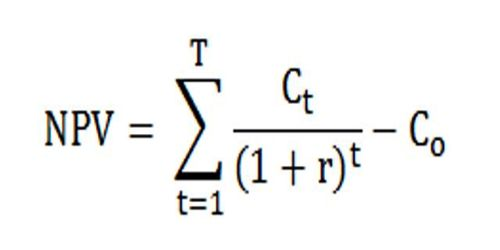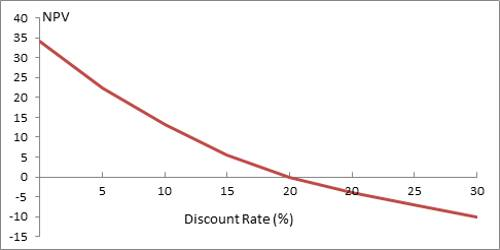# How Net Present Value (NPV) profiles are constructed?

Net Present Value (NPV) is the difference between the present value of cash inflows and the present value of cash outflows. NPV is used in capital budgeting to analyze the profitability of an investment or project.

The NPV calculation involves discounting all cash flows to the present based on an assumed discount rate. When the discount rate is large, there are larger differences between PV and FV (present and future value) for each cash flow than when the discount rate is small. Thus, when discount rates are large, cash flows further in the future affect NPV less than when the rates are small. Conversely, a low discount rate means that NPV is affected more by the cash flows that occur further in the future.

NPV is used to evaluate an investment assessment and give company management a plain way to tell if the investment will add value to the company.

The relationship between NPV and the discount rate used is calculated in a char called an NPV Profile. The independent variable is the discount rate and the dependent is the NPV. The NPV Profile assumes that all cash flows are discounted at the same rate.Fig: The NPV Profile graphs how NPV changes as the discount rate used changes

The NPV profile usually shows an inverse relationship between the discount rate and the NPV. While this is not necessarily true for all investments, it can happen because outflows generally occur before the inflows. A higher discount rate places more emphasis on earlier cash flows, which are generally the outflows. When the value of the outflows is greater than the inflows, the NPV is negative.

A special discount rate is highlighted in the IRR, which stands for Internal Rate of Return. It is the discount rate at which the NPV is equal to zero. And it is the discount rate at which the value of the cash inflows equals the value of the cash outflows.

The modified IRR has a significant advantage over the regular IRR like –

(a) MIRR has solved the reinvestment rate assumption of IRR. MIRR assumes that cash flows from all projects are reinvested at the cost of capital, while the regular IRR assumes that cash flows from each project are reinvested at the projects own IRR.

(b) MIRR also eliminates multiple IRR problems.

(c) The kinds of conflicts we encountered between NPV and the regular IRR will not occur in the case of MIRR.